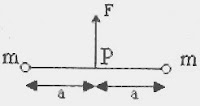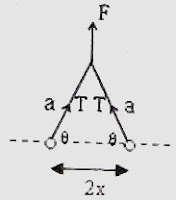## Thursday, August 02, 2007

### IIT-JEE 2007 Questions on Newton’s Laws of Motion

The following MCQ which appeared in the IIT-JEE question paper is interesting:Two particles of mass 'm' each are tied at the ends of a light string of length 2a. The whole system is kept on a frictionless horizontal surface with the string held tight so that each mass is at a distance 'a' from the centre P (as shown in the figure). Now, the mid point of the string is pulled vertically upwards with a small but constant force F. As a result, the particles move towards each other on the surface. The magnitude of acceleration, when the separation between them becomes 2x is
(a) Fa/2m√(a2–x2) (b) Fx/2m√(a2–x2)
(c)
Fx/2ma (d) F√(a2–x2) /2mxWith reference to the figure, F = 2T sinθ so that T = F/2sinθ where 'T' is the tension in the string

The horizontal force on each particle is T cosθ = Fcosθ/2sinθ = F/2tanθ.

Therefore, the magnitude of acceleration of each particle is given by

acceleration = force/mass = F/(2m tanθ) = F/[2m√(a2–x2)/x] = Fx/2m√(a2–x2)

The following Assertion-Reason Type Multiple Choice Question also appeared in the IIT-JEE 2007 question paper:

STATEMENT-1

A cloth covers a table. Some dishes are kept on it. The cloth can be pulled out without dislodging the dishes from the table

because

STATEMENT-2

For every action there is an equal and opposite reaction.

(a) Statement-1 is True, Statement-2 is True; Statement-2 is a correct explanation for Statement-1

(b) Statement-1 is True, Statement-2 is True; Statement-2 is NOT a correct explanation for Statement-1

(c) Statement-1 is True, Statement-2 is False

(d) Statement-1 is False, Statement-2 is True

This is a simple question which you can answer in no time if you have understood the property of inertia. In fact, by repetition this question has become a ‘knowledge’ type even though it is supposed to be an ‘understanding and application’ type.

If you pull the cloth out abruptly in the horizontal direction, the dishes will remain intact on the table. Because of inertia, the dishes cannot move sideways immediately and will touch the bare surface of the table where they will rest as before.

Statement-2 is Newton’s third law which is not the reason for statement-1.

So, the correct option is (b).

1.2.